Principal ideal

(diff) ← Older revision | Latest revision (diff) | Newer revision → (diff)

An ideal (of a ring, algebra, semi-group, or lattice) generated by one element, i.e. the smallest ideal containing the element.

The left principal ideal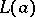of a ringcontains, in addition to the elementitself, also all the elementsthe right principal idealcontains all the elements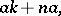and the two-sided principal ideal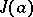contains all elements of the formwhere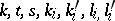are arbitrary elements ofand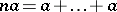(terms,). Ifis a ring with a unit element, the term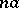may be omitted. In particular, for an algebraover a field,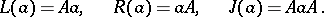In a semi-groupone also has left, right and two-sided ideals generated by an element, and they are equal, respectively, to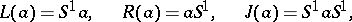where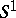is the semi-group coinciding withifcontains a unit, and is otherwise obtained fromby external adjunction of a unit.

The principal ideal of a latticegenerated by an elementis identical with the set of allsuch that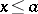; it is usually denoted by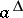,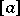, or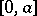if the lattice has a zero. Thus,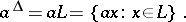In a lattice of finite length all ideals are principal.

Letbe an integral domain with field of fractions. A principal fractional ideal ofis an-submodule ofof the formfor some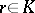.
Letbe a lattice. Dual to the principal ideal generated byone has the principal dual ideal or principal filter determined by, which is the set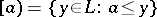. The principal ideal indetermined byis also denoted (more accurately) by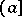.
A partially ordered set is a complete lattice if and only if it has a zero and every ideal inis principal.